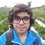# Pre-RMO 2014/3

Let $ABCD$ be a convex quadrilateral with perpendicular diagonals. If $AB = 20, BC = 70,$ and $CD = 90$, then what is the value of $DA$?

This note is part of the set Pre-RMO 2014Note by Pranshu Gaba
6 years, 8 months ago

This discussion board is a place to discuss our Daily Challenges and the math and science related to those challenges. Explanations are more than just a solution — they should explain the steps and thinking strategies that you used to obtain the solution. Comments should further the discussion of math and science.

When posting on Brilliant:

• Use the emojis to react to an explanation, whether you're congratulating a job well done , or just really confused .
• Ask specific questions about the challenge or the steps in somebody's explanation. Well-posed questions can add a lot to the discussion, but posting "I don't understand!" doesn't help anyone.
• Try to contribute something new to the discussion, whether it is an extension, generalization or other idea related to the challenge.
• Stay on topic — we're all here to learn more about math and science, not to hear about your favorite get-rich-quick scheme or current world events.

MarkdownAppears as
*italics* or _italics_ italics
**bold** or __bold__ bold
- bulleted- list
• bulleted
• list
1. numbered2. list
1. numbered
2. list
Note: you must add a full line of space before and after lists for them to show up correctly
paragraph 1paragraph 2

paragraph 1

paragraph 2

[example link](https://brilliant.org)example link
> This is a quote
This is a quote
    # I indented these lines
# 4 spaces, and now they show
# up as a code block.

print "hello world"
# I indented these lines
# 4 spaces, and now they show
# up as a code block.

print "hello world"
MathAppears as
Remember to wrap math in $$ ... $$ or $ ... $ to ensure proper formatting.
2 \times 3 $2 \times 3$
2^{34} $2^{34}$
a_{i-1} $a_{i-1}$
\frac{2}{3} $\frac{2}{3}$
\sqrt{2} $\sqrt{2}$
\sum_{i=1}^3 $\sum_{i=1}^3$
\sin \theta $\sin \theta$
\boxed{123} $\boxed{123}$

## Comments

Sort by:

Top Newest

Let the diagonals meet at a point $E$, and let $EA=a, EB=b, EC=c, ED=d$

Applying PT, we get

$a^{2}+b^{2}=20^{2} \rightarrow Eq.1$

$b^{2}+c^{2}=70^{2} \rightarrow Eq.2$

$c^{2}+d^{2}=90^{2} \rightarrow Eq.3$

Eq.3 - Eq.2

$d^{2}-b^{2}=90^{2} -70^{2}\rightarrow Eq.4$

Eq.1 + Eq.4

$a^{2}+d^{2}=90^{2} -70^{2}+20^{2}$

$\Rightarrow a^{2}+d^{2}=3600=AD^{2}$

$\Rightarrow AD=60$

- 6 years, 8 months ago

Log in to reply

60

- 6 years, 8 months ago

Log in to reply

Its $\boxed{60}$

- 5 years, 10 months ago

Log in to reply

60

- 6 years, 8 months ago

Log in to reply

60

- 6 years, 8 months ago

Log in to reply

60

- 6 years, 1 month ago

Log in to reply

60

- 6 years, 8 months ago

Log in to reply

just a small correction a^2+b^2= 20^2

- 6 years, 6 months ago

Log in to reply

Thnx edited.

- 6 years, 6 months ago

Log in to reply

60

- 5 years, 10 months ago

Log in to reply

Ans underroot 140

- 6 years, 8 months ago

Log in to reply

Only an integer answer is possible.

- 6 years, 8 months ago

Log in to reply

×

Problem Loading...

Note Loading...

Set Loading...